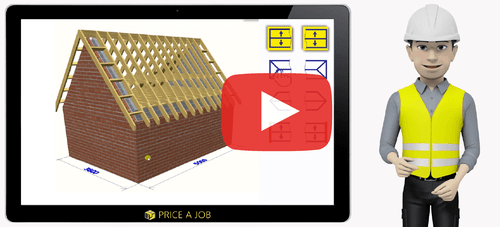8 Effective Estimation Techniques for Construction Businesses16/08/2018

One of the hardest things to do correctly in the construction process is to come up with an accurate project estimate. If you estimate the price too high, the bid may well go to someone else. Estimate too low and you'll wish it had as you'll be out of pocket and paying for things that your client should be. Budgeting for a project depends on the correct analysis early on.

Let's take a look at some of the estimation techniques that will enable you to get the estimate as accurate as possible when using estimating software.

1.Analogous Estimating

Also known as top-down estimation, this looks at previous project data in order to create new estimates for new projects. You'll need to have an archive of older and similar projects to compare.

2.Bottom-Up Estimating

Also commonly referred to as analytical estimating, this type of estimation divides the project up into a number of work packages made up of a series of tasks. This technique then estimates the cost of completing each task which are then added up to calculate the total cost of the project. This is one of the more accurate techniques, but can also be very time-consuming.

3.Parametric Estimating

This technique is best used on projects that involve repetitive tasks. The project is divided into units of work, and the cost of each unit is then determined. By multiplying the cost per unit by the total number of units you can get an estimate for the project. If the cost per unit ends up being correct, this technique can be pretty accurate.

4.Empirical Costing Methods

This is a great choice for projects that are similar. With this form of estimation data is taken from previous projects and used to calculate an estimated cost. Thanks to estimating software this technique is accurate and timely.

5.Expert Judgement

This technique is normally carried out by specialists who have worked on a wide variety and number of construction projects in the past. The expert will look at a past project and compare it to the current project in order to come up with a cost estimate. Whilst this technique doesn't come up with an exact number as it is a rough estimate, it is normally used to see if a project is feasible or not.

6.Three-Point Estimating

This method is a statistical one that uses formulae to come up with estimated costs by analysing optimistic, pessimistic and most likely estimates. This is an accurate technique providing the initial estimates are accurate.

7.Fixed Price Estimate

In a fixed price estimate the client agrees to pay a fixed price regardless of the amount of effort that goes into the project. This type of estimate is great if there is little uncertainty in the project. As the client you carry the risk of getting the project completed under the price agreed.

8.Three Point Estimation

This involves taking three of any of the above estimations and then making an average to get the total price.

System requirements